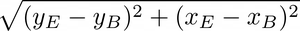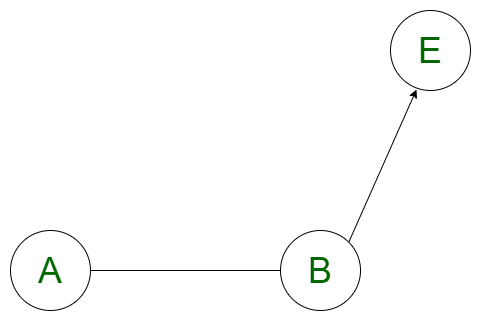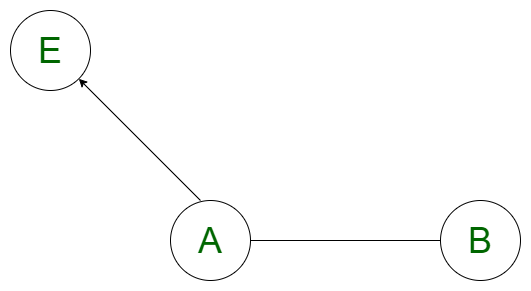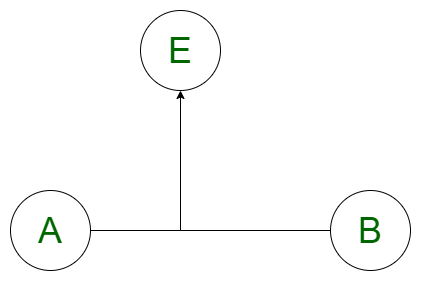Minimum distance from a point to the line segment using Vectors

• Difficulty Level : Easy
• Last Updated : 01 Jun, 2021

Given the coordinates of two endpoints A(x1, y1), B(x2, y2) of the line segment and coordinates of a point E(x, y); the task is to find the minimum distance from the point to line segment formed with the given coordinates.
Note that both the ends of a line can go to infinity i.e. a line has no ending points. On the other hand, a line segment has start and endpoints due to which length of the line segment is fixed.
Examples:

Input: A = {0, 0}, B = {2, 0}, E = {4, 0}

Output:
To find the distance, dot product has to be found between vectors AB, BE and AB, AE.
AB = (x2 – x1, y2 – y1) = (2 – 0, 0 – 0) = (2, 0)
BE = (x – x2, y – y2) = (4 – 2, 0 – 0) = (2, 0)
AE = (x – x1, y – y1) = (4 – 0, 0 – 0) = (4, 0)
AB . BE = (ABx * BEx + ABy * BEy) = (2 * 2 + 0 * 0) = 4
AB . AE = (ABx * AEx + ABy * AEy) = (2 * 4 + 0 * 0) = 8
Therefore, nearest point from E to line segment is point B.
Minimum Distance = BE == 2
Input: A = {0, 0}, B = {2, 0}, E = {1, 1}
Output:

Approach: The idea is to use the concept of vectors to solve the problem since the nearest point always lies on the line segment. Assuming that the direction of vector AB is A to B, there are three cases that arise:

1. The nearest point from the point E on the line segment AB is point B itself if the dot product of vector AB(A to B) and vector BE(B to E) is positive where E is the given point. Since AB . BE > 0, the given point lies in the same direction as the vector AB is and the nearest point must be B itself because the nearest point lies on the line segment.2. The nearest point from the point E on the line segment AB is point A itself if the dot product of vector AB(A to B) and vector BE(B to E) is negative where E is the given point. Since AB . BE < 0, the given point lies in the opposite direction of the line segment AB and the nearest point must be A itself because the nearest point lies on the line segment.3. If the dot product is 0, then the point E is perpendicular to the line segment AB and the perpendicular distance to the given point E from the line segment AB is the shortest distance. If some arbitrary point F is the point on the line segment which is perpendicular to E, then the perpendicular distance can be calculated as |EF| = |(AB X AE)/|AB||Below is the implementation of the above approach:

C++

 // C++ implementation of the approach #include   // To store the point #define Point pair #define F first #define S second using namespace std;   // Function to return the minimum distance // between a line segment AB and a point E double minDistance(Point A, Point B, Point E) {       // vector AB     pair AB;     AB.F = B.F - A.F;     AB.S = B.S - A.S;       // vector BP     pair BE;     BE.F = E.F - B.F;     BE.S = E.S - B.S;       // vector AP     pair AE;     AE.F = E.F - A.F,     AE.S = E.S - A.S;       // Variables to store dot product     double AB_BE, AB_AE;       // Calculating the dot product     AB_BE = (AB.F * BE.F + AB.S * BE.S);     AB_AE = (AB.F * AE.F + AB.S * AE.S);       // Minimum distance from     // point E to the line segment     double reqAns = 0;       // Case 1     if (AB_BE > 0) {           // Finding the magnitude         double y = E.S - B.S;         double x = E.F - B.F;         reqAns = sqrt(x * x + y * y);     }       // Case 2     else if (AB_AE < 0) {         double y = E.S - A.S;         double x = E.F - A.F;         reqAns = sqrt(x * x + y * y);     }       // Case 3     else {           // Finding the perpendicular distance         double x1 = AB.F;         double y1 = AB.S;         double x2 = AE.F;         double y2 = AE.S;         double mod = sqrt(x1 * x1 + y1 * y1);         reqAns = abs(x1 * y2 - y1 * x2) / mod;     }     return reqAns; }   // Driver code int main() {     Point A = make_pair(0, 0);     Point B = make_pair(2, 0);     Point E = make_pair(1, 1);       cout << minDistance(A, B, E);       return 0; }

Java

 // Java implementation of the approach class GFG {   static class pair {     double F, S;     public pair(double F, double S)     {         this.F = F;         this.S = S;     }     public pair() {     } }   // Function to return the minimum distance // between a line segment AB and a point E static double minDistance(pair A, pair B, pair E) {       // vector AB     pair AB = new pair();     AB.F = B.F - A.F;     AB.S = B.S - A.S;       // vector BP     pair BE = new pair();     BE.F = E.F - B.F;     BE.S = E.S - B.S;       // vector AP     pair AE = new pair();     AE.F = E.F - A.F;     AE.S = E.S - A.S;       // Variables to store dot product     double AB_BE, AB_AE;       // Calculating the dot product     AB_BE = (AB.F * BE.F + AB.S * BE.S);     AB_AE = (AB.F * AE.F + AB.S * AE.S);       // Minimum distance from     // point E to the line segment     double reqAns = 0;       // Case 1     if (AB_BE > 0)     {           // Finding the magnitude         double y = E.S - B.S;         double x = E.F - B.F;         reqAns = Math.sqrt(x * x + y * y);     }       // Case 2     else if (AB_AE < 0)     {         double y = E.S - A.S;         double x = E.F - A.F;         reqAns = Math.sqrt(x * x + y * y);     }       // Case 3     else     {           // Finding the perpendicular distance         double x1 = AB.F;         double y1 = AB.S;         double x2 = AE.F;         double y2 = AE.S;         double mod = Math.sqrt(x1 * x1 + y1 * y1);         reqAns = Math.abs(x1 * y2 - y1 * x2) / mod;     }     return reqAns; }   // Driver code public static void main(String[] args) {     pair A = new pair(0, 0);     pair B = new pair(2, 0);     pair E = new pair(1, 1);       System.out.print((int)minDistance(A, B, E)); } }   // This code is contributed by 29AjayKumar

Python3

 # Python3 implementation of the approach from math import sqrt   # Function to return the minimum distance # between a line segment AB and a point E def minDistance(A, B, E) :       # vector AB     AB = [None, None];     AB = B - A;     AB = B - A;       # vector BP     BE = [None, None];     BE = E - B;     BE = E - B;       # vector AP     AE = [None, None];     AE = E - A;     AE = E - A;       # Variables to store dot product       # Calculating the dot product     AB_BE = AB * BE + AB * BE;     AB_AE = AB * AE + AB * AE;       # Minimum distance from     # point E to the line segment     reqAns = 0;       # Case 1     if (AB_BE > 0) :           # Finding the magnitude         y = E - B;         x = E - B;         reqAns = sqrt(x * x + y * y);       # Case 2     elif (AB_AE < 0) :         y = E - A;         x = E - A;         reqAns = sqrt(x * x + y * y);       # Case 3     else:           # Finding the perpendicular distance         x1 = AB;         y1 = AB;         x2 = AE;         y2 = AE;         mod = sqrt(x1 * x1 + y1 * y1);         reqAns = abs(x1 * y2 - y1 * x2) / mod;           return reqAns;   # Driver code if __name__ == "__main__" :       A = [0, 0];     B = [2, 0];     E = [1, 1];       print(minDistance(A, B, E));   # This code is contributed by AnkitRai01

C#

 // C# implementation of the approach using System;   class GFG {   class pair {     public double F, S;     public pair(double F, double S)     {         this.F = F;         this.S = S;     }     public pair() {     } }   // Function to return the minimum distance // between a line segment AB and a point E static double minDistance(pair A, pair B, pair E) {       // vector AB     pair AB = new pair();     AB.F = B.F - A.F;     AB.S = B.S - A.S;       // vector BP     pair BE = new pair();     BE.F = E.F - B.F;     BE.S = E.S - B.S;       // vector AP     pair AE = new pair();     AE.F = E.F - A.F;     AE.S = E.S - A.S;       // Variables to store dot product     double AB_BE, AB_AE;       // Calculating the dot product     AB_BE = (AB.F * BE.F + AB.S * BE.S);     AB_AE = (AB.F * AE.F + AB.S * AE.S);       // Minimum distance from     // point E to the line segment     double reqAns = 0;       // Case 1     if (AB_BE > 0)     {           // Finding the magnitude         double y = E.S - B.S;         double x = E.F - B.F;         reqAns = Math.Sqrt(x * x + y * y);     }       // Case 2     else if (AB_AE < 0)     {         double y = E.S - A.S;         double x = E.F - A.F;         reqAns = Math.Sqrt(x * x + y * y);     }       // Case 3     else     {           // Finding the perpendicular distance         double x1 = AB.F;         double y1 = AB.S;         double x2 = AE.F;         double y2 = AE.S;         double mod = Math.Sqrt(x1 * x1 + y1 * y1);         reqAns = Math.Abs(x1 * y2 - y1 * x2) / mod;     }     return reqAns; }   // Driver code public static void Main(String[] args) {     pair A = new pair(0, 0);     pair B = new pair(2, 0);     pair E = new pair(1, 1);       Console.Write((int)minDistance(A, B, E)); } }   // This code is contributed by 29AjayKumar

Javascript



Output:

1

Time Complexity: O(1 )

Auxiliary Space: O(1)

My Personal Notes arrow_drop_up
Recommended Articles
Page :